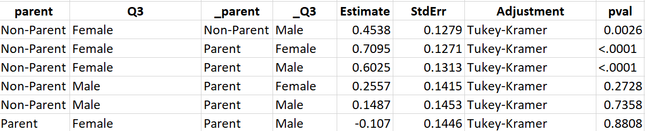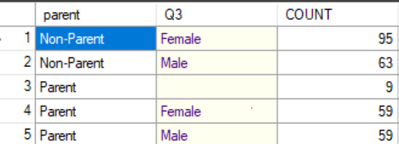## How to get n values for these?

Hello everyone,

I have the below output. I would also want to have N values for these. How can we produce them for all 6 rows?

``````ods output Diffs = AP_3way_diffs(keep = parent _parent _q3 q3 estimate stderr adjustment adjp rename=(adjp=pval));
proc mixed data=fixptsex;
class parent Q3;
model maleprod_n = parent Q3  parent*Q3;
run;``````

My output from this was as below:I would also like to have individual N values for each row.

Thanks

1 ACCEPTED SOLUTION

Accepted Solutions

## Re: How to get n values for these?

Not counting the cell where Q3 is missing ... you have four different combinations, and those are the N values.

The PROC MIXED output gives the differences between the LSMEANS, that is 6 differences (Four things taken two at a time is 4*3/2 = 6). There isn't really an N for the differences of LSMEANS in this case, but since you have the PROC FREQ output, you can indicate somehow how many data points are in each combination of the original variables.

--
Paige Miller
3 REPLIES 3

## Re: How to get n values for these?

You want this ??

``````proc FREQ data=fixptsex;
tables parent * Q3 / missing list;
run;
/* end of program */``````

Koen

## Re: How to get n values for these?

This is the freq by q3 and parent.
It is not including maleprod or cross tabluation by q3*parent q3*parent. How would I also include but just get 6 obs.

I tried the code you provided. It's giving me as below:## Re: How to get n values for these?

Not counting the cell where Q3 is missing ... you have four different combinations, and those are the N values.

The PROC MIXED output gives the differences between the LSMEANS, that is 6 differences (Four things taken two at a time is 4*3/2 = 6). There isn't really an N for the differences of LSMEANS in this case, but since you have the PROC FREQ output, you can indicate somehow how many data points are in each combination of the original variables.

--
Paige Miller
Discussion stats
• 3 replies
• 424 views
• 1 like
• 3 in conversation